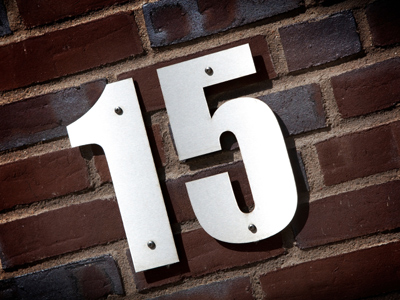5 x 3 = 15.

# 3 Times Table

Learning the times tables is a vital tool when multiplying numbers. By the end of Year 2 children are often fluent in their times tables up to 10. When they enter into KS2 in Year 3 they will also be taught some higher times tables in their Maths and Numeracy lessons. By the end of KS2 they will be familiar with all of the times tables from one to twelve.

Learning the 3 times table involves multiplying numbers by 3. Knowing your times tables helps with all kinds of maths. Even if you get all the questions right first time keep on taking the quiz as you can improve on the time it takes you to answer all the questions. Learning your times tables will make multiplying numbers, and so your maths lessons, a lot easier!

Test your knowledge of the 3 times table by playing this quiz.

1.
12 x 3 =
12
30
36
15
2.
9 x 3 =
12
19
26
27
To multiply a number by 9 just times it by 10 then take it away, so 3 x 10 = 30, 30 - 3 = 27
3.
7 x 3 =
21
23
25
30
4.
3 x 3 =
3
6
8
9
A number multiplied by itself is known as a square number, so 9 is 3 squared
5.
5 x 3 =
10
15
18
22
15 is 3 + 3 + 3 + 3 + 3 and 5 + 5 + 5
6.
8 x 3 =
11
20
24
26
There are 24 hours in a day
7.
6 x 3 =
9
18
22
24
8.
4 x 3 =
10
12
14
15
9.
11 x 3 =
15
33
14
35
10.
2 x 3 =
3
5
6
9
To times a number by 2 just double it